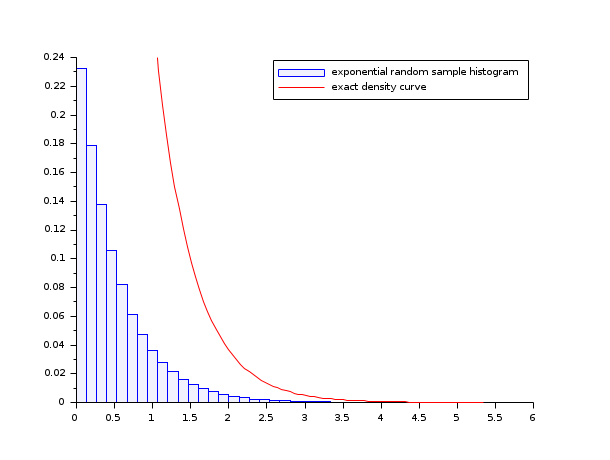Scilab Home page | Wiki | Bug tracker | Forge | Mailing list archives | ATOMS | File exchange
Please login or create an account
Change language to: Français - Português - 日本語 - Русский

Please note that the recommended version of Scilab is 6.1.0. This page might be outdated.
See the recommended documentation of this function

Scilab Help >> Graphics > 2d_plot > histplot

# histplot

plot a histogram

### Syntax

```[cf, ind] = histplot(n, data [,normalization] [,polygon], <opt_args>)
[cf, ind] = histplot(x, data [,normalization] [,polygon], <opt_args>)```

### Arguments

n

positive integer (number of classes)

x

increasing vector defining the classes (`x` may have at least 2 components)

data

vector (data to be analysed)

normalization

a boolean (%t (default value) or %f)

polygon

a boolean (%t or %f (default value))

<opt_args>

This represents a sequence of statements `key1=value1,key2=value2` ,... where `key1`, `key2,...` can be any optional plot2d parameter (`style,strf,leg, rect,nax, logflag,frameflag, axesflag` ).

cf

This represents a sequence of statements `key1=value1,key2=value2` ,... where `key1`, `key2,...` can be any optional plot2d parameter (`style,strf,leg, rect,nax, logflag,frameflag, axesflag` ).

ind

This represents a sequence of statements `key1=value1,key2=value2` ,... where `key1`, `key2,...` can be any optional plot2d parameter (`style,strf,leg, rect,nax, logflag,frameflag, axesflag` ).

### Description

This function plots a histogram of the `data` vector using the classes `x`. When the number `n` of classes is provided instead of `x`, the classes are chosen equally spaced and x(1) = min(data) < x(2) = x(1) + dx < ... < x(n+1) = max(data) with dx = (x(n+1)-x(1))/n.

The classes are defined by C1 = [x(1), x(2)] and Ci = ( x(i), x(i+1)] for i >= 2. Noting Nmax the total number of `data` (Nmax = length(data)) and Ni the number of `data` components falling in Ci, the value of the histogram for x in Ci is equal to Ni/(Nmax (x(i+1)-x(i))) when `normalization` is true (default case) and else, simply equal to Ni. When normalization occurs the histogram verifies:when x(1)<=min(data) and max(data) <= x(n+1)

Any plot2d (optional) parameter may be provided; for instance to plot a histogram with the color number 2 (blue if std colormap is used) and to restrict the plot inside the rectangle [-3,3]x[0,0.5], you may use `histplot(n,data, style=2, rect=[-3,0,3,0.5])`.

Frequency polygon is a line graph drawn by joining all the midpoints of the top of the bins of a histogram. Therefore we can use `histplot` function to plot a polygon frequency chart.

The optional argument `polygon` connects the midpoint of the top of each bar of a histogram with straight lines.

If `polygon=%t` we will have a histogram with frequency polygon chart.

Enter the command `histplot()` to see a demo.

### Examples

• Example #1: variations around a histogram of a gaussian random sample
```d=rand(1,10000,'normal');  // the gaussian random sample
clf(); histplot(20,d)
clf(); histplot(20,d,normalization=%f)
clf(); histplot(20,d,leg='rand(1,10000,''normal'')',style=5)
clf(); histplot(20,d,leg='rand(1,10000,''normal'')',style=16, rect=[-3,0,3,0.5]);```• Example #2: histogram of a binomial (B(6,0.5)) random sample
```d = grand(1000,1,"bin", 6, 0.5);
c = linspace(-0.5,6.5,8);
clf()
subplot(2,1,1)
histplot(c, d, style=2)
xtitle("Normalized histogram")
subplot(2,1,2)
histplot(c, d, normalization=%f, style=5)
xtitle("Non normalized histogram")```• Example #3: histogram of an exponential random sample
```lambda = 2;
X = grand(100000,1,"exp", 1/lambda);
Xmax = max(X);
clf()
histplot(40, X, style=2)
x = linspace(0,max(Xmax),100)';
plot2d(x,lambda*exp(-lambda*x),strf="000",style=5)
legend(["exponential random sample histogram" "exact density curve"]);```• Example #4: the frequency polygon chart and the histogram of a gaussian random sample
```n=10;
data=rand(1,1000,"normal");
clf(); histplot(n, data, style=12, polygon=%t);
legend(["normalized histogram" "frequency polygon chart"]);```### See also

• histc — computes an histogram
• hist3d — 3D representation of a histogram
• plot2d — 2D plot
• bar — bar histogram
• dsearch — distribute, locate and count elements of a matrix or hypermatrix in given categories

### Comments

Add a comment:
Please login to comment this page.

 Report an issue << graypolarplot 2d_plot paramfplot2d >>

 Scilab EnterprisesCopyright (c) 2011-2017 (Scilab Enterprises)Copyright (c) 1989-2012 (INRIA)Copyright (c) 1989-2007 (ENPC)with contributors Last updated:Tue Feb 14 15:02:50 CET 2017